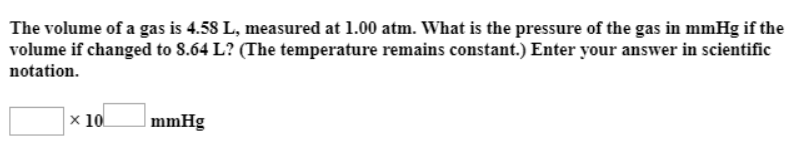# Solution: The volume of a gas is 4.58 L, measured at 1.00 atm. What is the pressure of the gas in mmHg if the volume if changed to 8.64 L? (The temperature remains constant.) Enter your answer in scientific notation.

###### Problem

The volume of a gas is 4.58 L, measured at 1.00 atm. What is the pressure of the gas in mmHg if the volume if changed to 8.64 L? (The temperature remains constant.) Enter your answer in scientific notation.View Complete Written Solution